# 3 Digit Subtraction without Regrouping

3 Digit Subtraction without Regrouping – Question 1

Subtract the following: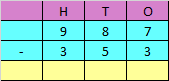Explanation

Subtracting the digits at ones place

7 – 3 = 4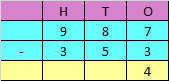Subtracting the digits at tens place

8 – 5 = 3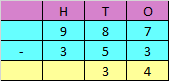Subtracting the digits at hundreds place

9 – 3 = 6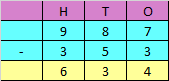Hence, the difference is 634

3 Digit Subtraction without Regrouping – Question 2

Subtract the following: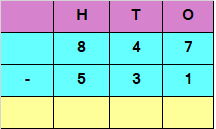Explanation

Subtracting the digits at ones place

7 – 1 = 6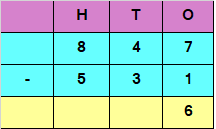Subtracting the digits at tens place

4 – 3 = 1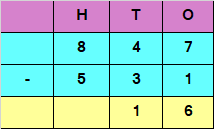Subtracting the digits at hundreds place

8 – 5 = 3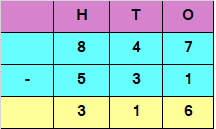Hence, the difference is 316